# Module 4: MATH AND DATA ANALYSIS

## Learning Objectives

After reading this lesson, you should be able to:

• solve linear equations in MATLAB,

• solve nonlinear equations in MATLAB,

• manipulate polynomials and polynomial coefficients,

• plot data with the syms data type.

## How do I solve for roots of a linear equation?

In this section, we will go over using MATLAB to solve for the roots of an equation using solve(). For example, $$x-2=0$$ has a root at $$x=2$$.

Example 1 shows the procedure for solving for the root of a linear equation in MATLAB. Note that when the equation is defined in Example 1, we write y = 9*x +7 == 10. This means that the equation 9*x + 7 == 10 is stored in the variable y, which we call subsequently in the program like we would any other variable. The right-hand side of the equation that you enter does not have to be equal to zero. For example, you could also write 9*x == 3 and still get the same answer from MATLAB.

You might be asking at this point, “Why do we need double equals when entering the equation?” The simple answer to this is that MATLAB needs to recognize that you are entering an equation rather than storing something in a variable. Remember that y = 9*x + 7 == 10 has two parts. Entering the equation 9*x + 7 == 10 is stored in the variable y. MATLAB needs a way to differentiate between these two operations. All you need to remember is to put the double equals (==) when entering an equation. MATLAB will return an error if you fail to do so.

### Example 1

Solve for the root of the $$9x+7=10$$.Display the equation and the root of the equation using a single fprintf() function.

Solution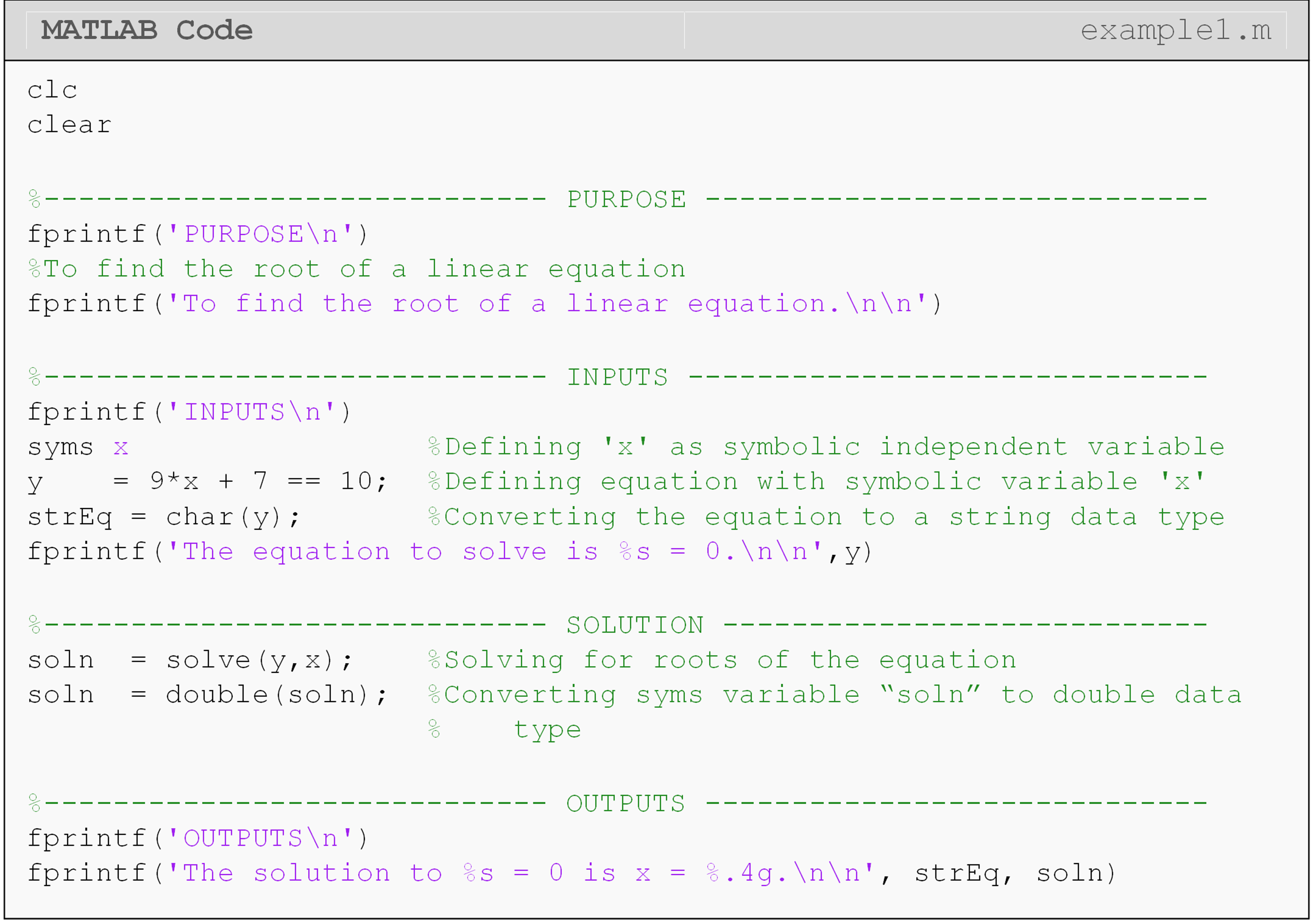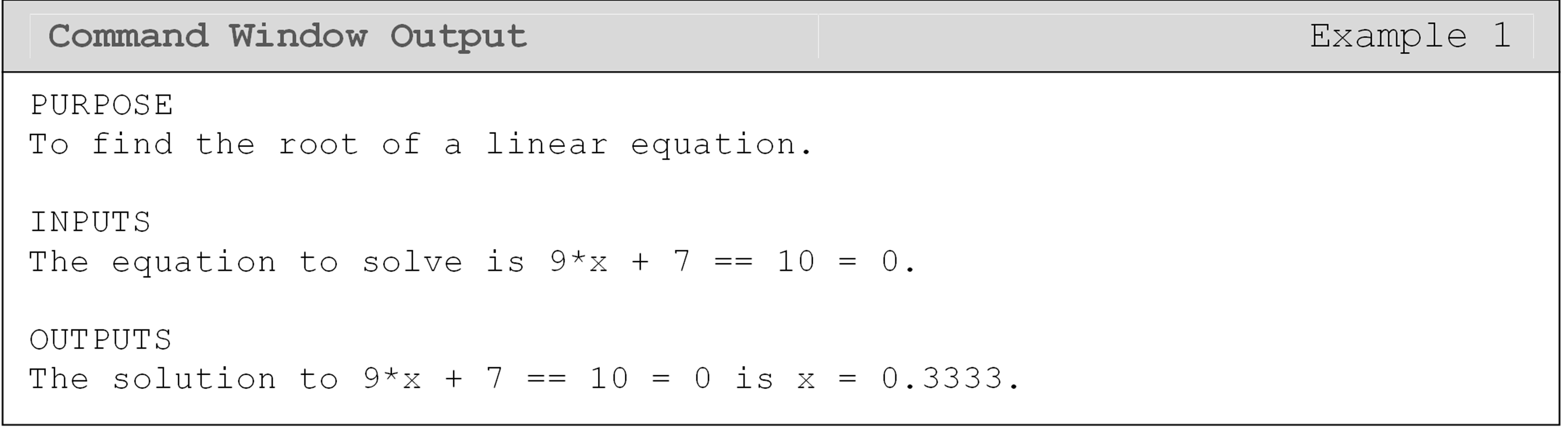## What is a nonlinear equation?

A nonlinear equation is an equation that has nonlinear terms of the unknowns. One of the simplest examples of a nonlinear equation is the quadratic equation that has the form

$$ax^{2} + bx + c = 0$$ (1)

where,

$$a \neq 0$$.

The values of a, b, and c are constant coefficients and x is the independent variable. If one were to solve a nonlinear equation using traditional methods they would need to be provided at least two items: the nonlinear equation and the unknown variable to solve for.

Take the case of the equation given in the above Equation (1). The nonlinear equation is a quadratic equation and the variable to solve for is x. This example can be solved using the widely known solution of a quadratic equation as

$$x = \displaystyle\frac{- b \pm \sqrt{b^{2} - 4ac}}{2a}$$ (2)

However, other more complex nonlinear equations can take longer to solve exactly, while most nonlinear equations are impossible to solve analytically. Another example of a nonlinear equation is $$\text{sin}(2x) = \displaystyle\frac{3x}{7}$$. In these cases, MATLAB can be used to solve the nonlinear equations or even to verify the results obtained from an analytical method.

## How can I use MATLAB to solve nonlinear equations?

As we know, MATLAB is a tool. Just like a mechanic might use a wrench to solve a problem, an engineer, scientist or mathematician might use MATLAB to solve a problem. In the case of solving a nonlinear equation, MATLAB can be used to solve nearly any equation (limited more so by the operating computer than the program itself).

We used solve() to find the roots of a linear equation as a demonstration of symbolic variables. However, solve() is also used to find the roots of nonlinear equations. In this case, we may get multiple roots. Unique, none and infinite number of solutions are other possibilities for nonlinear equations. Multiple roots will be stored in vector form, which you can see in Example 2. The variable soln is a vector that holds the three roots we found from the third-order polynomial equation.

You can see in Example 2 that the solution to the nonlinear equation has three possible values. We know this is correct because the equation is a cubic equation. The outputs to the solve command are stored in a vector and can each be pulled out of the vector by using matrix references, which we covered in Lesson 2.6.

### Example 2

Solve the nonlinear equation $$x^3-15x^2+47x=33$$ for x using MATLAB (that is, find its roots).

Solution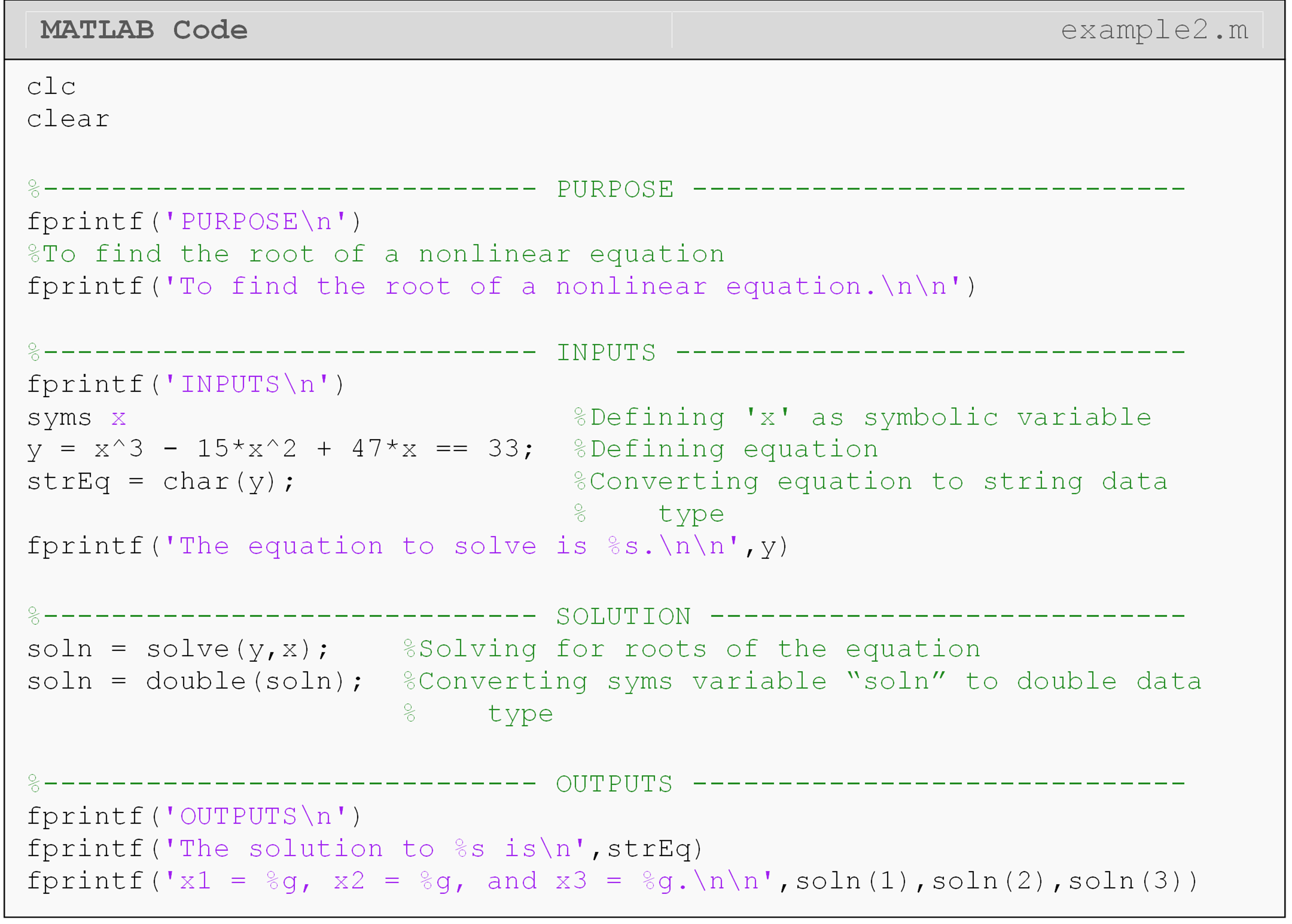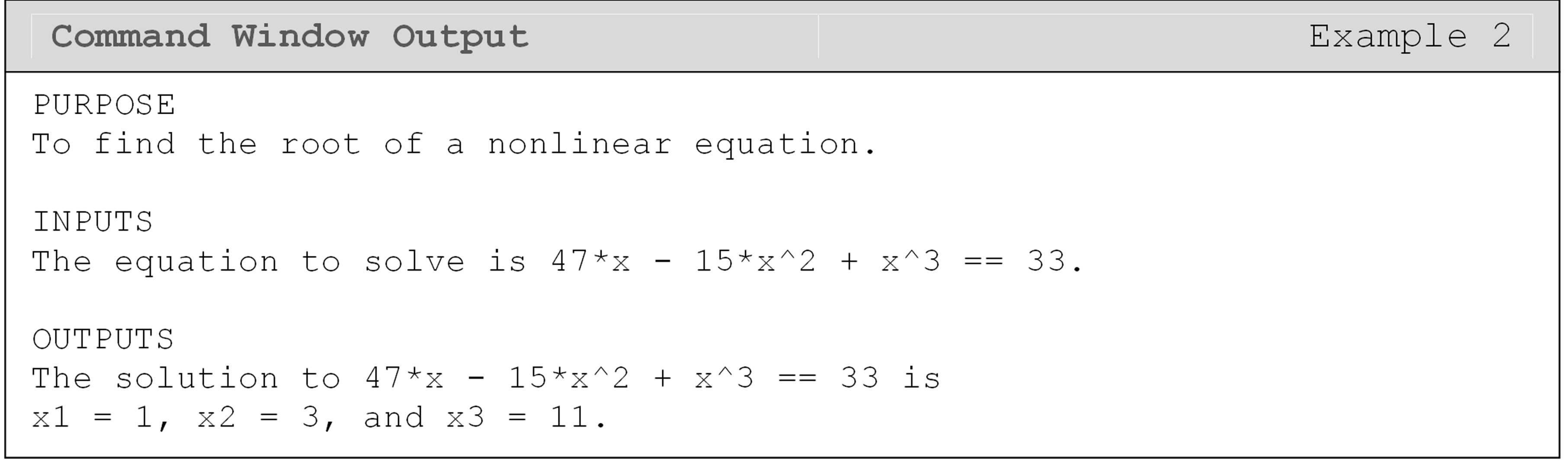MATLAB also provides a method to solve an equation numerically using vpasolve() (note that solve() uses analytical methods). Although polynomial equations have a finite number of solutions (which is equal to the order of the polynomial), other nonlinear equations can possibly have an infinite number of solutions (e.g., $$\text{tan}\left(x \right) = x$$). Therefore, MATLAB does not report all solutions in the same way. Check the vpasolve() documentation for specifics.

In Example 3, we show an application that shows a real-world problem. The only difference here is the prerequisite mathematical knowledge to set up the problem (find the appropriate equation). The programming portion is more or less the same, which is the beauty of MATLAB and many other similar programming languages. Once written, a program like the one in Example 2 can be quickly edited for a completely different equation like the one in Example 3. We chose to use the aforementioned vpasolve() in Example 3.

### Example 3

Find the depth x to which a ball is floating in water (see Figure 1) based on the following cubic equation

$4R^{3}S - 3x^{2}R = - x^{3}$

where,
R = radius of the ball,

S = specific gravity of the ball.

Use values of R and S to be 0.055 and 0.6, respectively.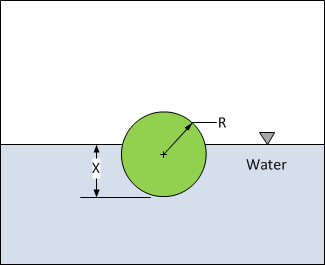Figure 1: Diagram of a partially submerged sphere in water for use with Example 3.

Solution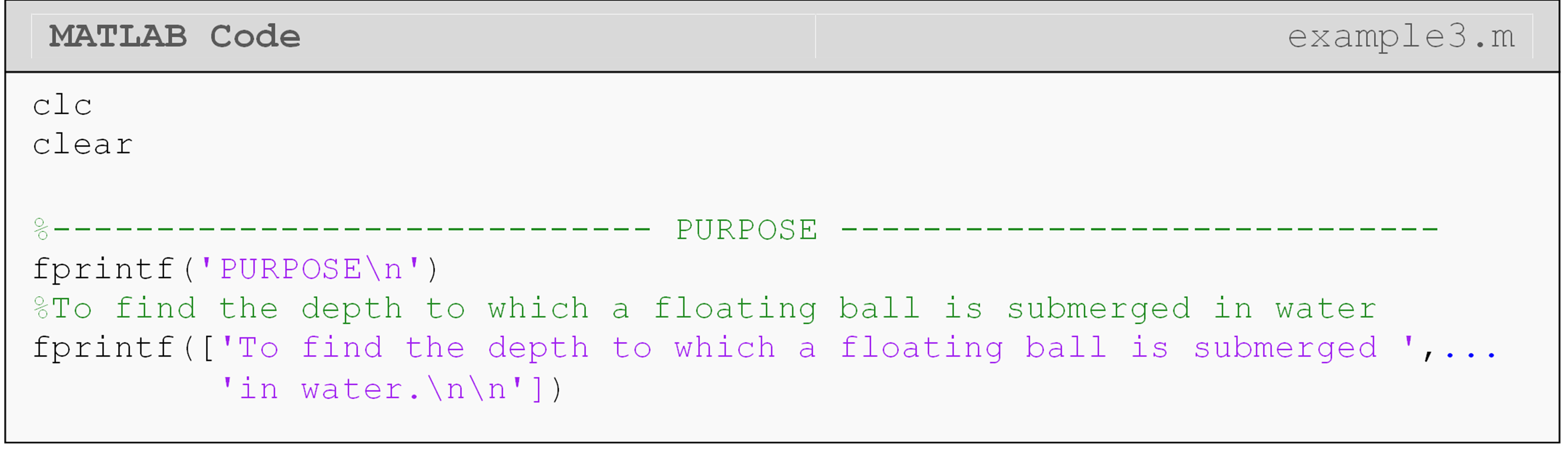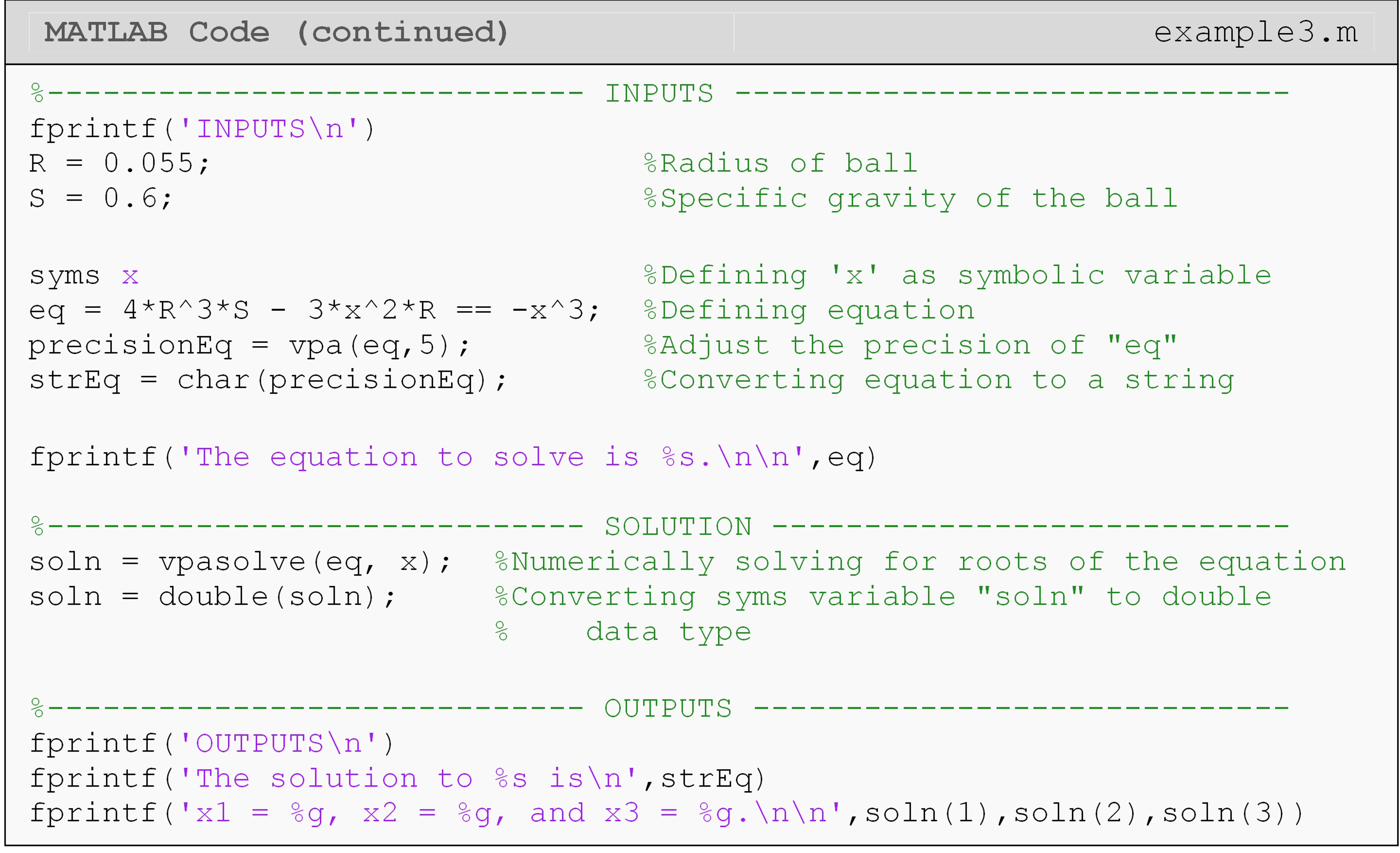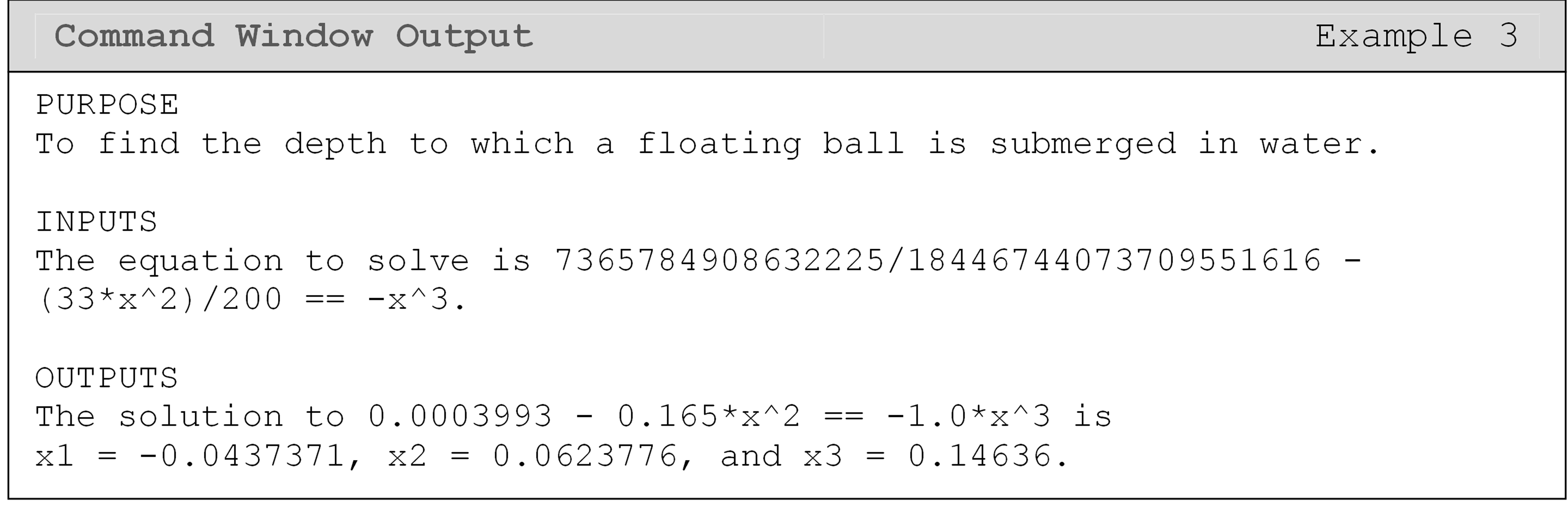Note the three possible solutions to the third order polynomial equation for Example 2. Since the limits of x are given by $$0 \leq x \leq 2R$$ and R = 0.055, the limits of x are $$0 \leq x \leq 0.11$$. Hence, the only physically acceptable solution is $$x = 0.0624$$.

## Is there a faster way to work with polynomial equations in MATLAB?

We can create a symbolic polynomial from a vector of coefficients using the function poly2sym(). (You can do the reverse operation with sym2poly().) This can be especially helpful when using functions that return a vector of coefficients directly such as polyfit(), which we will cover later in Lessons 4.7 and 4.8. You can see in Example 4 that the coefficient output (coefs) is not very intuitive. However, we can directly convert the coefficients to polynomial form, which is more intuitive, and find the roots of the corresponding polynomial with the roots() function.

### Example 4

Given any size vector of polynomial coefficients, generate the full polynomial in symbolic form and find its roots.

Test the program using the coefficient vector $$\left\lbrack \begin{matrix} 0.003 & -0.0748 & -4.2660 & 1.8370 \end{matrix} \right\rbrack$$.

Solution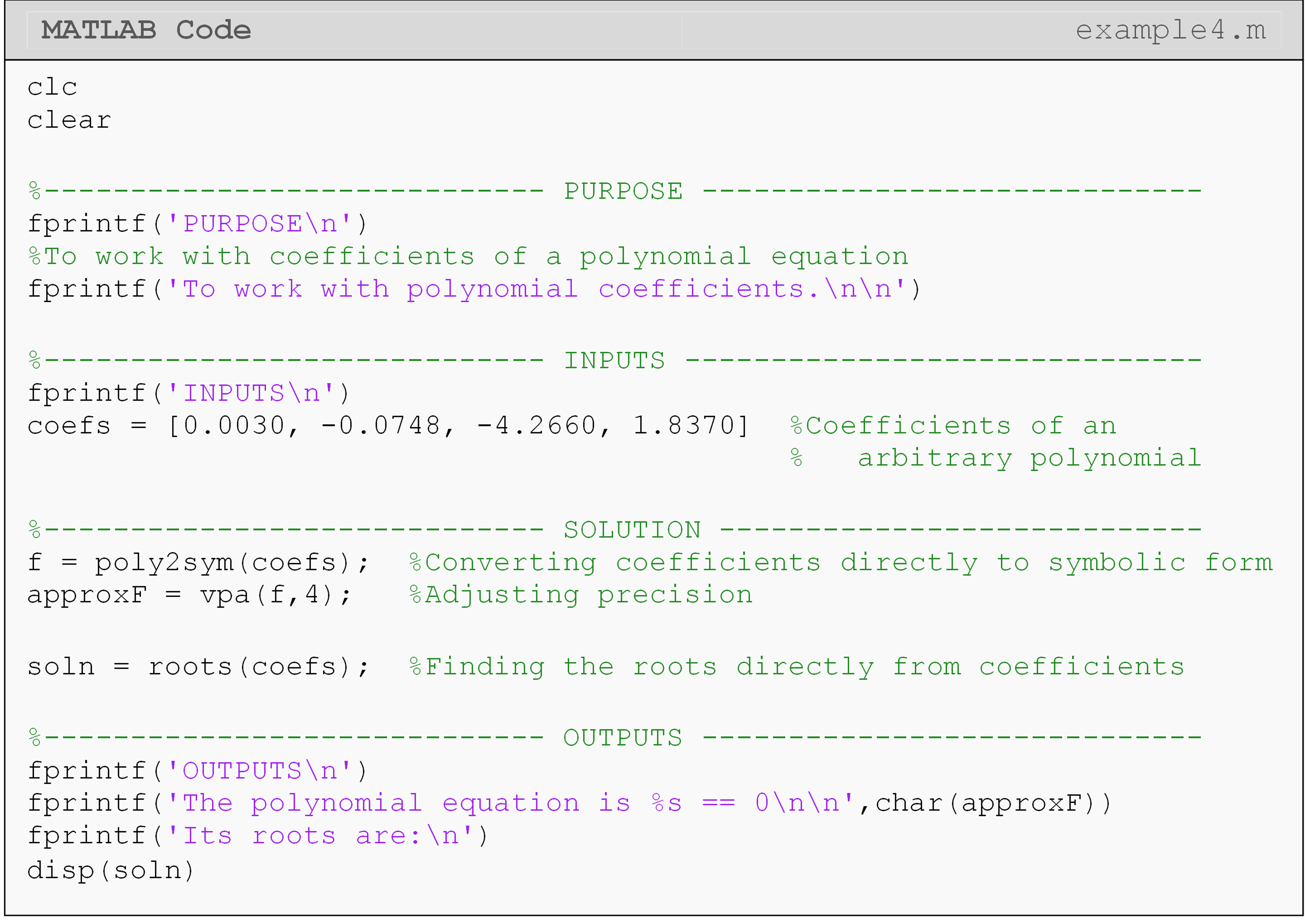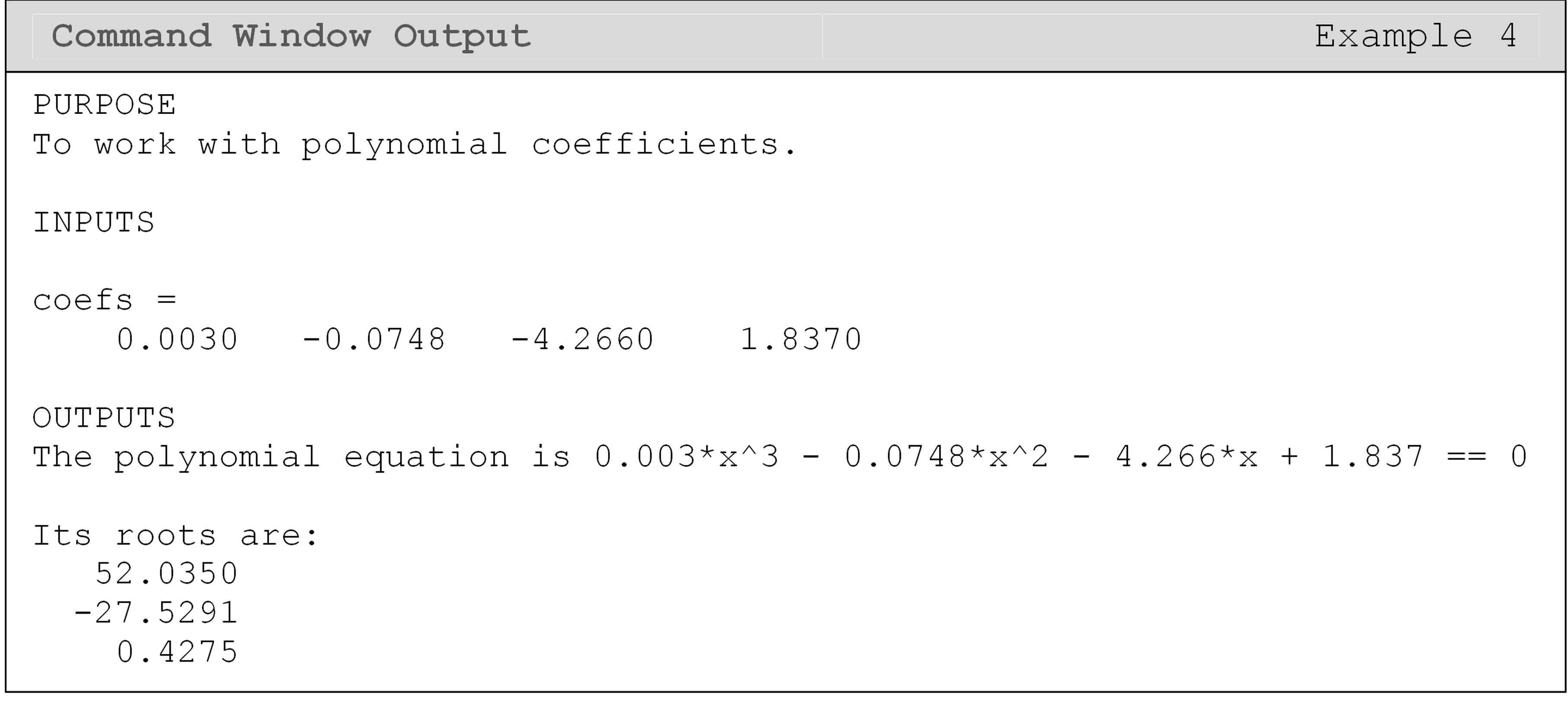## Can I plot with symbolic variables?

Plotting with syms has all the same considerations as any other function you wish to plot with the additional step of converting to a data type that is accepted by the function you are using to plot (e.g., plot(), [bar()](https://www.mathworks.com/help/matlab/ref/bar.html?s_tid=srchtitle), etc.). That is, you will likely need to convert from sym to a numeric data type to plot the data.

To get a vector of data points to plot, we can use the subs() function. Although it was used in the previous examples to replace a variable with just a single number or character, it also accepts vector inputs as the replacement value(s). When the input is a vector, the output is a corresponding vector as well.

### Example 5

Plot the function $$\displaystyle{y(x)=4x^3-20x^2+9x}$$ and its zeros on the same plot. Choose the domain to plot the function based upon the solutions found for the zeros of the function. Include title, axis labels, legend, and plot grid.

Solution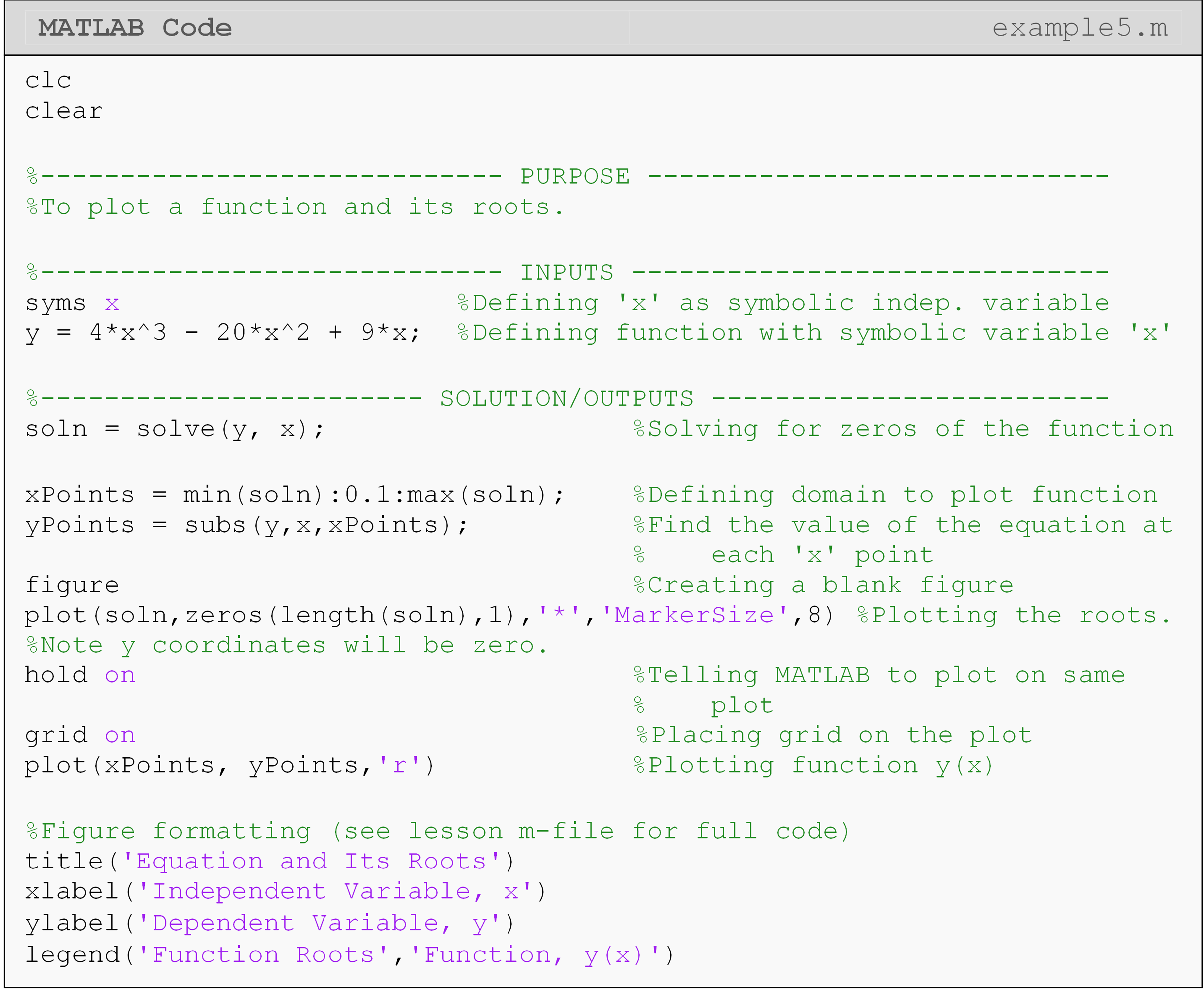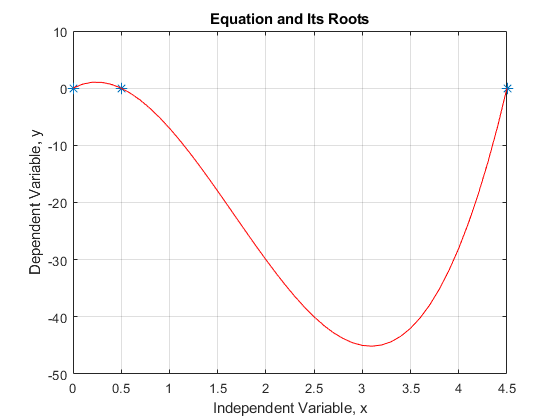Figure 6: The MATLAB figure output for Example 5.

## Lesson Summary of New Syntax and Programming Tools

Find the roots of an equation solve() solve(eq,x)
Numerically find the roots of an equation vpasolve() vpasolve(eq,x)
Form a symbolic polynomial function from coefficients poly2sym() poly2sym(coefs,x)
Extract the coefficients from a polynomial sym2poly() sym2poly(polynomial)
Get the roots of a polynomial using only is coefficients as an input roots() roots(coefs)

## Multiple Choice Quiz

(1). A MATLAB function for solving an equation analytically is

(a)  fsolved()

(b)  nonlinear()

(c)  vpasolve()

(d)  solve()

(2). The MATLAB function to find the solution to a polynomial equation given only its coefficients is

(a)  solve()

(b)  coefs()

(c)  roots()

(d)  polysolve()

(3). To solve $$x^2+2x=0$$, which line of code should be added to the program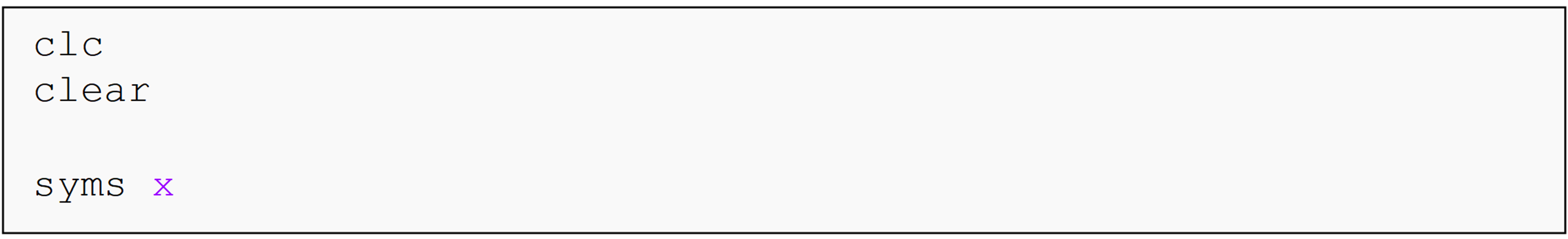(a)  solve(x^2+2x==0,x)

(b)  solve(x^2-2x,x)

(c)  solve(x^2+2*x=0,x)

(d)  solve(x^2+2*x==0,x)

(4). The MATLAB substitution function subs() has __________ input variables

(a)  1

(b)  2

(c)  3

(d)  4

(5). The output solutions for a single nonlinear equation given by the solve() function

(a)  gives only one solution to the equation.

(b)  gives only the physically acceptable solution(s).

(c)  provides the solutions as separate outputs.

(d)  stores solutions in a single vector.

## Problem Set

(1). Use the solve() function to find the solution of the equation $$3x^{2} + 2x = 5$$. Display the answers in the Command Window.

(2). Given that the value of the two variables a and b can be changed, set up the equation $$ax^{2} + 2x = b$$ so that it can be solved for any real values of a and b.

(3). Solve the nonlinear equation $$2x+e^{-x}=5$$. Output both the original equation and at least one of its roots using a single fprintf() function.

(4). You are working for ‘DOWN THE TOILET COMPANY Inc.’ that makes floats for H3H3 toilets. The floating ball has a specific gravity of 0.6 and has a radius of 5.5 cm. You are asked to find the depth to which the ball is submerged when floating in water (Figure A).

The equation that gives the depth x in meters to which the ball is submerged underwater is given by

$x^{3} - 0.165x^{2} + 3.993 \times 10^{- 4} = 0$

Find the depth, $$x$$, to which the ball is submerged underwater.Figure A: Floating ball in water for Exercise 4.

(5). You have a spherical storage tank containing oil (Figure B). The tank has a diameter of 6 ft. You are asked to calculate the height, h, to which a dipstick 8 ft long would be wet with oil when immersed in the tank when it contains 4 $$\text{f}\text{t}^{3}$$ of oil. The equation that gives the height, $$h$$, of the liquid in the spherical tank for the above-given volume and radius is given by

$f(h) = h^{3} - 9h^{2} + 3.8197 = 0$

Find the height, $$h$$, to which the dipstick is wet with oil.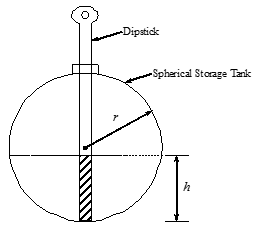Figure B: Dipstick inside spherical tank which contains some oil. Used in Exercise 5.

(6). You are making a cool bookshelf to carry your interesting books that range from $$8\frac12$$” to 11” in height. The bookshelf material is wood, which has a Young’s Modulus of $$3.667 \text{ Msi}$$, length (L) of 29”, a thickness of 3/8”, and width of 12”. You want to find the maximum vertical deflection of the bookshelf. The vertical deflection of the shelf is given by $v(x) = 0.42493\ \times \text{ 1}\text{0}^{- 4}x^{3} - 0.13533 \times \text{ 1}\text{0}^{- 8}x^{5} - 0.66722\ \times \text{ 1}\text{0}^{- 6}x^{4} - 0.018507x$

where $$x$$ is the position along the length of the beam (Figure C). To find the maximum deflection we need to find where

$f(x) = \frac{dv}{dx} = 0$

and conduct the second derivative test. The equation that gives the position,$$x$$, where the deflection is extreme (minimum or maximum) is given by $- 0.67665\ \times \text{ 1}\text{0}^{- 8}x^{4} - 0.26689\ \times \text{ 1}\text{0}^{- 5}x^{3} + 0.12748\ \times \text{ 1}\text{0}^{- 3}x^{2} - 0.018507 = 0$

(a)  Plot the deflection of the beam, $$v(x)$$, for values of x from 0 to L.

(b)  Find the position $$x$$ where the deflection is maximum.

(c)  Find the value of the maximum deflection.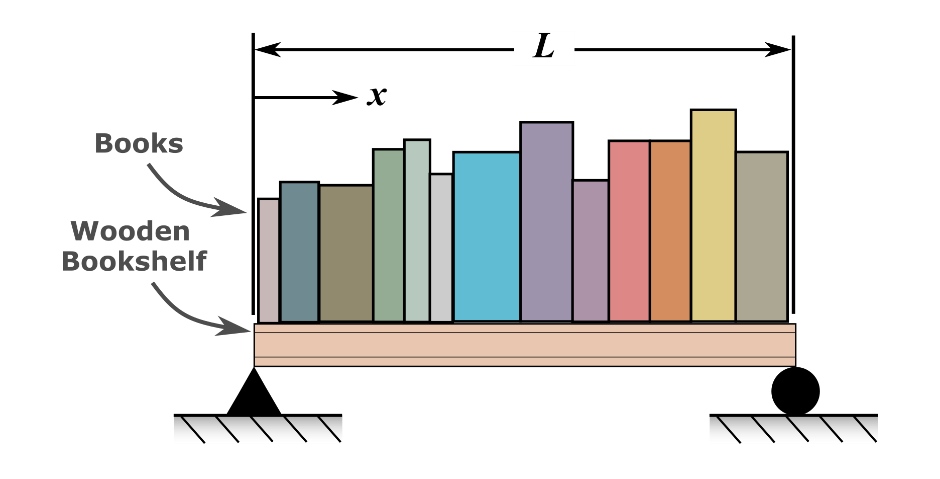Figure C: Schematic of bookshelf used in Exercise 6.

(d)  A trunnion has to be cooled from a temperature of $$80{^\circ}F$$before it is shrink-fitted into a steel hub (See Figure D on the next page). The equation that gives the temperature, $$T_{f}$$, to which the trunnion has to be cooled to obtain the desired contraction is given by

${f(T_{f}) = - 0.50598\ \times \text{ 1}\text{0}^{- \text{10}}T_{f}^{3} + 0.38292\ \times \text{ 1}\text{0}^{- 7}T_{f}^{2} + }{0.74363\ \times \text{ 1}\text{0}^{- 4}T_{f} + 0.88318\ \times \text{ 1}\text{0}^{- 2} = 0}$

Find the roots of the equation to find the temperature, $$T_{f}$$, to which the trunnion has to be cooled. Choose the physically acceptable root of the equation.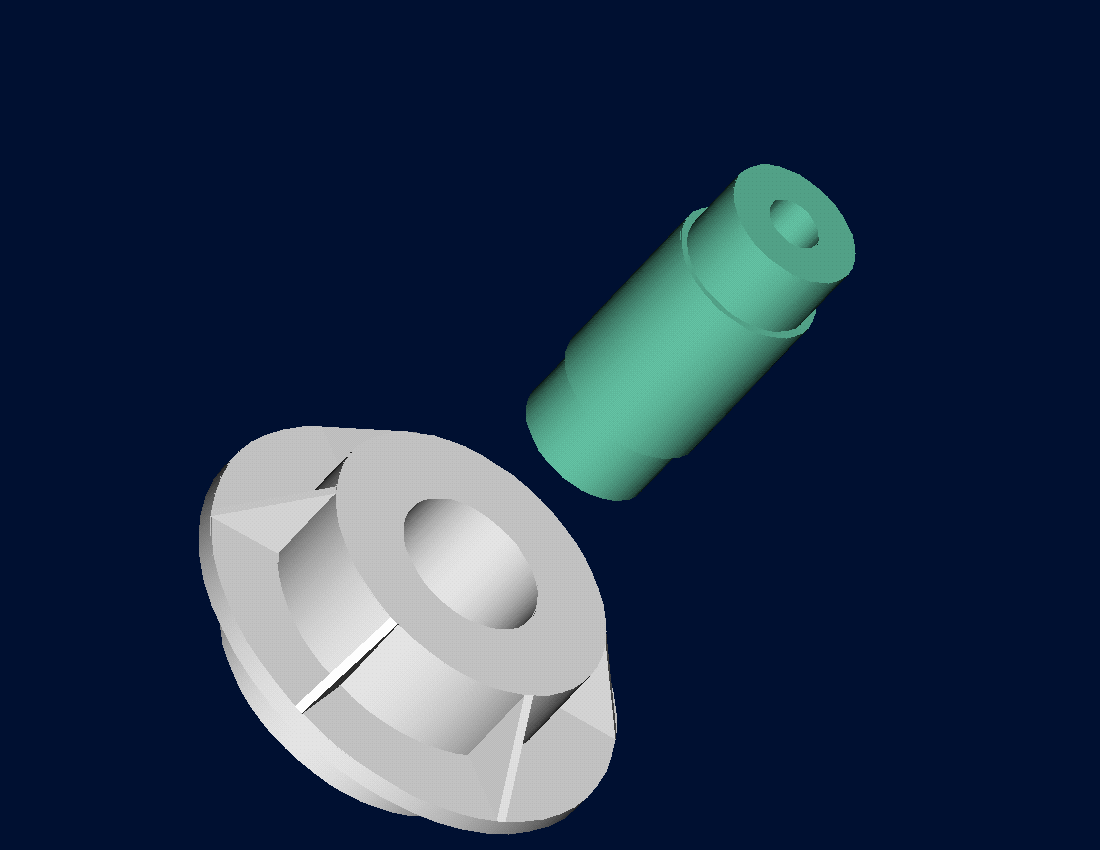Figure D: Trunnion and hub shown prior to shrink fitting for Exercise 7.